# Autonomous Raspberry Mini Car

Daniela Preto
Bruno Manica
Augusto Zwirtes

## Sumary

Implement a self-driving remote controlled car using image recognition with machine learning and neural networks. It will be two parts, in the first one the car will be capable of following a path on the floor and stop when it detect a obstacle in front of the car. In the last part, the car must be able to recognise road signs and obstacles.

## Motivation

Artificial intelligence is a hot subject and promising in all areas of science. One of our group members is currently working with image recognition and neural networks on her TCC. We intend to use that knowledge and apply it on a practical function that both other members are very interested into the subject of autonomous vehicles. Since it is a subject of heated ethical debates, our attempt will be to replicate its features, viability and main issues in order to be capable of having a better understanding of what is going on behind the steering wheel of a driverless vehicle.

## Goals

In order to be considered a driverless car our Mini Car has to be capable of recognizing a road, traffic signs, obstacles and be able to deviate from it , emergency stops, and accelerate accordingly, following basic traffic laws.

## Methodology

We will use the Raspberry Pi 3 board attached to a remote control car chassis. Programming a Raspberry Pi camera module connected to the board to get the current image of what is in front of the car. Integrate OpenCV image recognition libraries with TensorFlow Application. Teach a TensorFlow neural network to be able to recognize the path and the traffic signs and to know what to do in each case.
A motor microcontroller will be needed to accomplish proper energy deliverance to both engines (one to control the speedy and when the car should stop, and the other to control when the car should change direction).
We are going to use two separate power supply, for the board we choose a 10000mah power bank and to the microcontroller we choose to use a pack of 7,2v batteries. At the last part, the Raspberry Pi must be able to tell what the microcontroller should do.

## Tools

• Hardware:
• Raspberry Pi 3 Model B;
• H-Bridge Motor Driver L293D - Integrated circuit used for robotics, that enables a voltage to be applied across a load in either direction;
• Infrared Sensor - To capture the images in front of the car;
• Camera Module for Raspberry Pi;
• Stepper Motor - To control the front axle;
• DC motor - To control the rear axle;
• Resistors - Several will be used to control the voltage of peripheral components such as the infrared sensor and the dc motors
• Breadboard - Used to ease the construction of temporary electronic prototypes without soldering.
• Jumper Cables - Several will be used to connect each peripheral to the integrated circuit and the Raspberry GPIO.
• Software:
• TensorFlow API;
• OpenCV libraries;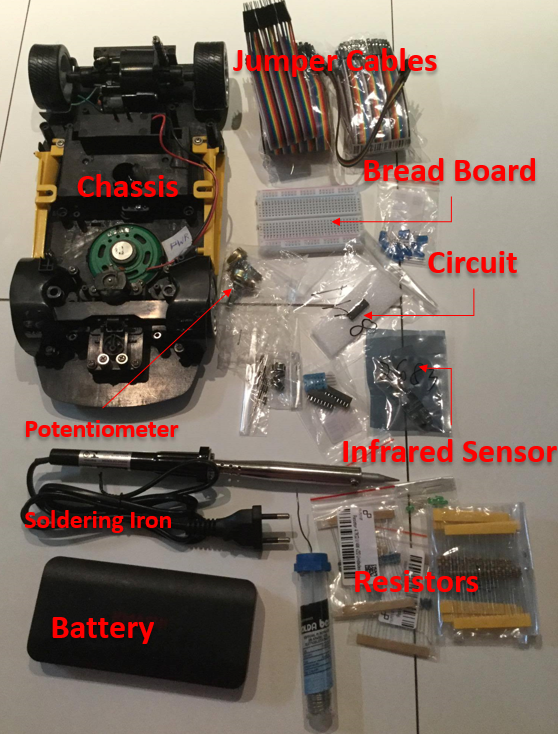T1: Detail project planning and review the literature.
T2: Demonstrate project viability.
T3:Connect microcontrollers, motors and all the hardwares parts to the Raspberry to be able to control what the car is going to do by a keyboard.
T4:Connect the camera to raspberry to be able to follow a line. Integrate a infrared sensor with the board.
(Optional)T5:Process the image using the OpenCV libraries and then teach the neural network to perform image recognition using TensorFlow Application.

## Deliverables

D1: Report of the detailed project planning and literature revised.
D2: A project viability report, containing a list of needed tools.
D3: Demonstration of the hardware working.
D4: A video showing the project working and a report containig everything that was done until the actual state.
D5: A video showing the project working and a report containig everything that was done in the whole.

## Schedule

Task 25/09 02/10 11/10 20/10 25/10 01/11 08/11 15/11 22/11 29/11
Task4 X X D4
Task5 X X D5

## OpenCV Viability

In order to make this project viable, we had to install and configure OpenCV in our Raspberry Pi. This library in the board doesn’t work the same way as in desktops because Raspberrypi doesn’t use Opencv camera interface. Due to this fact, we had to install and configure raspicam, which is a library that access the camera and interfaces with OpenCV, that will processes the images reading by raspicam.
Installing OpenCV on the system wasn’t straightforward at all. First, we had to follow some tutorials, and several additional libraries were needed to be installed on Raspberry, below there is the history of libraries installed through apt-get, until we could run OpenCV on Raspberry Pi.

• libffmpeg-dev;
• libjpeg-dev;
• libavformat-edv;
• libavcodec-dev;
• libjpeg-dev;
• libxvidcore-dev;
• libx264-dev;
• libav-tools;
• libjasper-dev;
• libswscale;
• libswscale-dev;
• libv4l-dev;
• libtiff5-dev;
• libpng12-dev;
• libavresample-dev;
• libgstreamer1.0-dev;
• libgtk2.0-dev.

Afterwards, OpenCV was installed and ran using Raspberry camera.

## Raspberry Pi Camera

A 5-megapixel camera model was chosen to use in this project. We were able to connect to the Raspberry Pi and record images.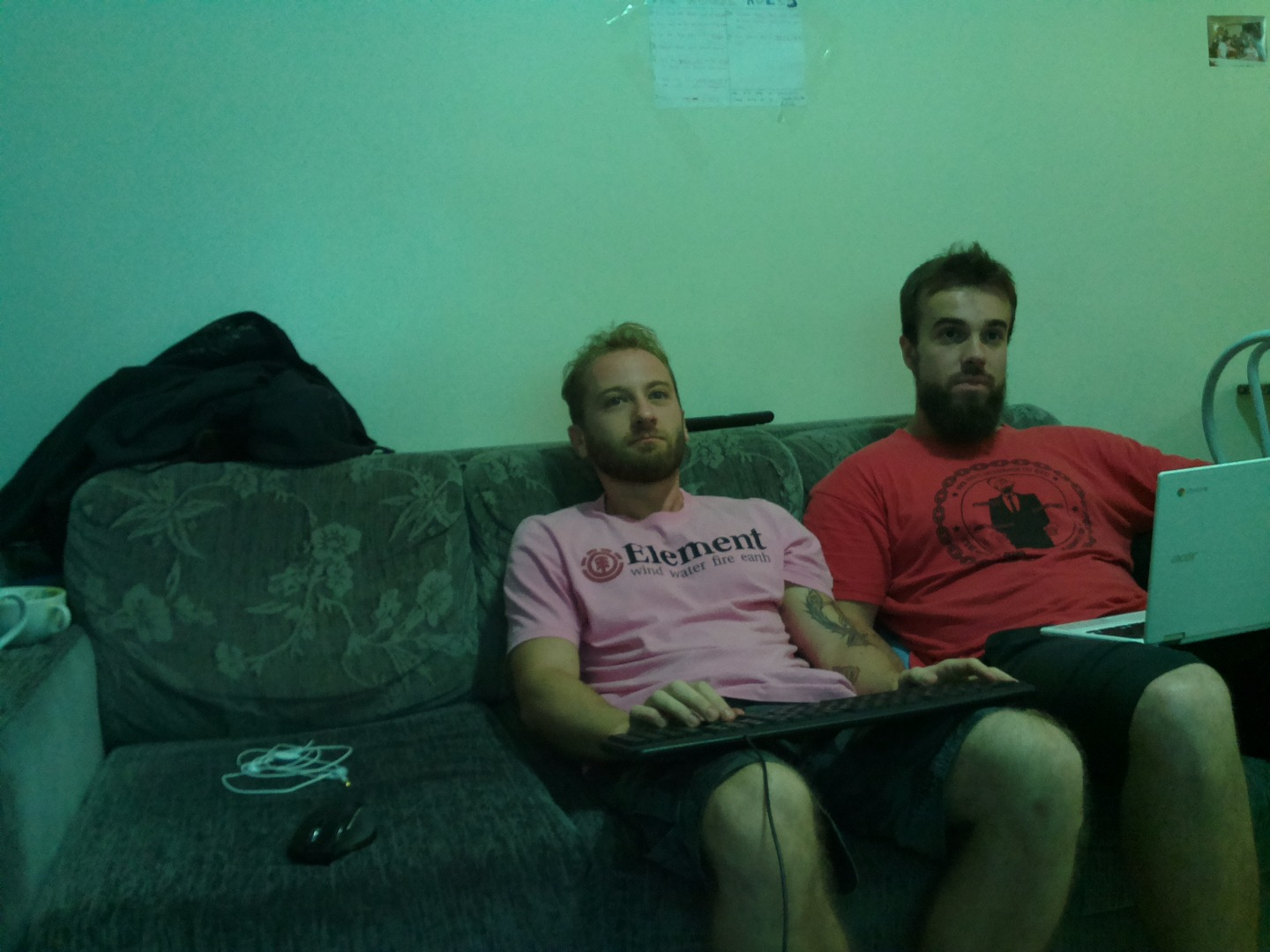(Photo taken with Raspberry Pi Camera.)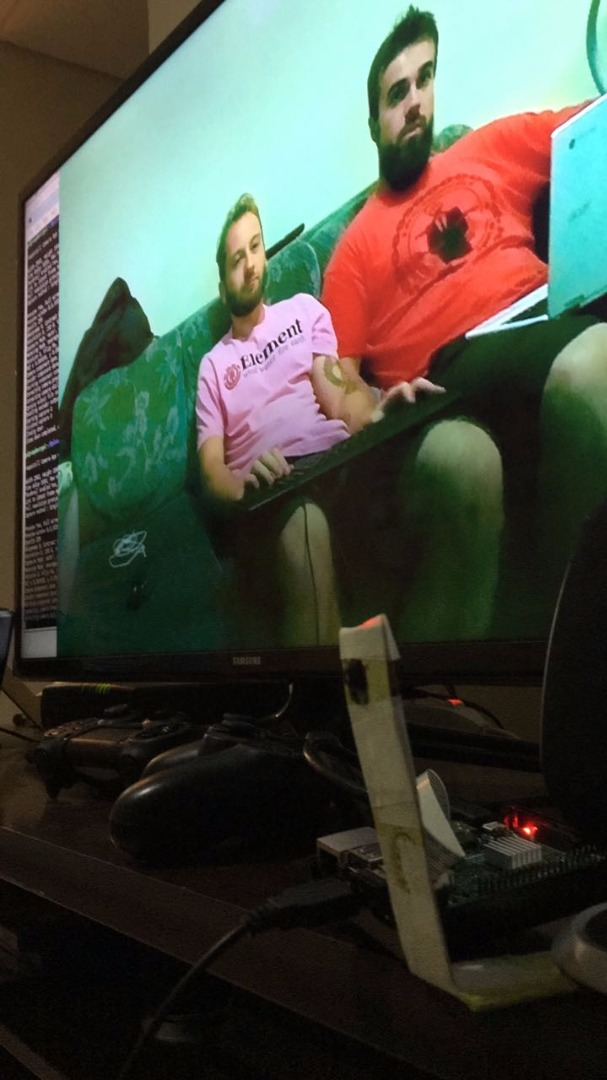(Photo taken with a cellphone showing the same photo above.)

## L293D - Integrated Circuit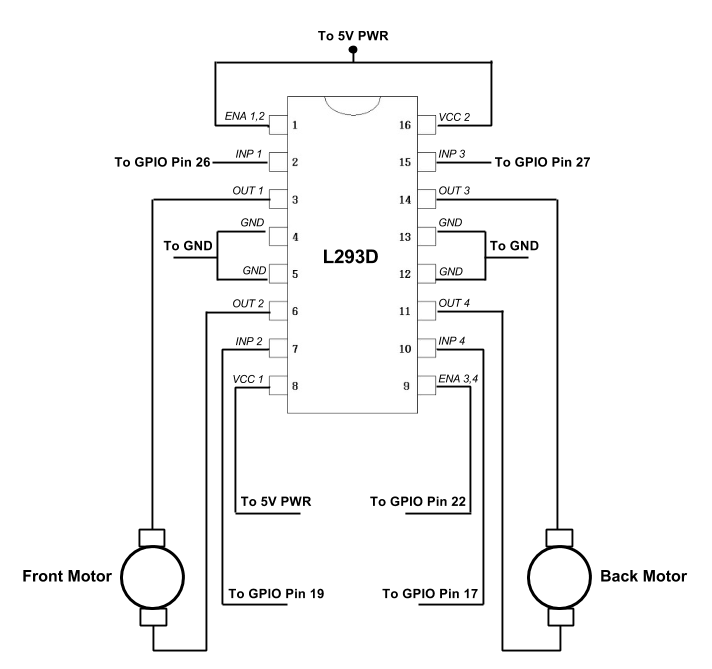The approach we used to control and test the motors was using a high level language(python). In order to the motors work correctly, we had to use the Broadcom SOC channel (BCM) numbers instead of the Raspberry physical pin numbers, as the stantard GPIO libraries used them to enumerate the pins. The L293D has four inputs and four outputs to control motors.
The inputs are connected to the physical pin 11(BCM 17) and physical pin 13 (BCM 27) to control the back motor, and physical pin 35(BCM 19) and physical pin 37 (BCM 26) to control the front motor. Two outputs are set to the back motor and two to the front motor.

## Keyboard Control

Three classes were developed to control the cart with the keyboard.
The Main Class:

```import tkinter as tk
import os
from pi_vector import Vector
from getpass import getuser

isRpi = getuser() == "pi"
if isRpi:
from controller import Controller

class MainWindow(tk.Tk):
''' Handles the keyboard events and calculates the direction '''
def __init__(self):
tk.Tk.__init__(self)
#Configure key bindings and data structures
self.direction = Vector(0, 0)
self.controller = Controller()
self.bind("<Escape>", lambda _ : self.quit())
self.bind("<FocusOut>", self.clear_direction)
#self.bind("<KeyPress-Space>", lambda _ : self.controller.horn(True))
#self.bind("<KeyRelease-Space>", lambda _ : self.controller.horn(False))
self.keys = {"Up" : Vector(0, 1),
"Down" : Vector(0, -1),
"Right" : Vector(1, 0),
"Left" : Vector(-1, 0)}
for n in self.keys:
self.bind("<KeyPress-" + n + ">", self.keydown)
self.bind("<KeyRelease-" + n + ">", self.keyup)
# Build window
self.title("Raspberry pi autonomous car")
self.width = 300
self.height = 200
self.label_height = 40
self.geometry("{}x{}".format(self.width, self.height))
self.columnconfigure(0, minsize=self.width)
self.rowconfigure(0, minsize=self.height - self.label_height)
self.rowconfigure(1, minsize=self.label_height)
#Build widgets
self.label = tk.Label(self, text="Use the direction keys in your\nkeyboard to move the car",
font=("Helvetica", 10), anchor=tk.CENTER);
self.shape = None
self.canvas = tk.Canvas(self, width=self.width, height=self.height - self.label_height)
center_x, center_y = self.width / 2., (self.height - self.label_height) / 2.
self.canvas.create_oval(center_x - 10, center_y - 10, center_x + 10, center_y + 10, fill="red")
# Draw the widgets
self.canvas.grid(column = 0, row=0, sticky=tk.N+tk.S+tk.E+tk.W)
self.label.grid(column=0, row=1, sticky=tk.N+tk.S+tk.E+tk.W)
self.after(100, self.check_collision)

def clear_direction(self, e):
self.direction = Vector(0, 0)
self.update()

def update(self):
def translate(p, v): # when drawing in the GUI the y axis is inverted
return Vector(p.x + v.x, p.y - v.y)

if self.shape is not None:
self.canvas.delete(self.shape)

center = Vector(self.width / 2., (self.height - self.label_height) / 2.)
d = translate(center, self.direction.normal() * 50)
self.shape = self.canvas.create_line(center.x, center.y, d.x, d.y, width=10, fill="red")
#sends the direction vector to the controller
self.controller << self.direction

def keydown(self, e):
self.direction += self.keys[e.keysym]
self.update()

def keyup(self, e):
self.direction -= self.keys[e.keysym]
self.update()

def check_collision(self):
self.controller.check_collision()
self.after(100, self.check_collision)

def main():
os.system("xset r off")
try:
win = MainWindow()
win.focus_set()
win.mainloop()
os.system("xset r on")
except:
os.system("xset r on")

if __name__ == '__main__':
main()```

The Controller class (It is connected to the pins of the board to know which direction to follow)

```import RPi.GPIO as GPIO
from time import sleep

BACK_MOTOR_DATA_ONE = 17
BACK_MOTOR_DATA_TWO = 27
BACK_MOTOR_ENABLE_PIN = 18
FRONT_MOTOR_DATA_ONE = 19
FRONT_MOTOR_DATA_TWO = 26
INITIAL_PWM_DUTY_CYCLE = 100
PWM_FREQUENCY = 1000
COLLISION_PIN = 2
HORN_FREQUENCY = 500

class Controller:

def __init__(self):
self.collided = False
GPIO.setmode(GPIO.BCM)
GPIO.setup(BACK_MOTOR_DATA_ONE, GPIO.OUT)
GPIO.setup(BACK_MOTOR_DATA_TWO, GPIO.OUT)
GPIO.setup(FRONT_MOTOR_DATA_ONE, GPIO.OUT)
GPIO.setup(FRONT_MOTOR_DATA_TWO, GPIO.OUT)
GPIO.setup(COLLISION_PIN, GPIO.IN, pull_up_down=GPIO.PUD_UP)
GPIO.setup(BACK_MOTOR_ENABLE_PIN, GPIO.OUT)
self._pwm = GPIO.PWM(BACK_MOTOR_ENABLE_PIN, PWM_FREQUENCY)
self._pwm.start(INITIAL_PWM_DUTY_CYCLE)

def __lshift__(self, dir):
self.go_to(dir)

def go_to(self, dir):
if dir.size > 0:
if dir.y == 0:
self.back_idle()
elif dir.x == 0:
self.front_idle()

if dir.y > 0:
self.forward()
elif dir.y < 0:
self.reverse()

if dir.x > 0:
self.right()
elif dir.x < 0:
self.left()
else:
self.stop()

def forward(self):
if not self.collided:
GPIO.output(BACK_MOTOR_DATA_ONE, True)
GPIO.output(BACK_MOTOR_DATA_TWO, False)

def reverse(self):
GPIO.output(BACK_MOTOR_DATA_ONE, False)
GPIO.output(BACK_MOTOR_DATA_TWO, True)

def left(self):
GPIO.output(FRONT_MOTOR_DATA_ONE, True)
GPIO.output(FRONT_MOTOR_DATA_TWO, False)

def right(self):
GPIO.output(FRONT_MOTOR_DATA_ONE, False)
GPIO.output(FRONT_MOTOR_DATA_TWO, True)

def stop(self):
self.back_idle()
self.front_idle()

def front_idle(self):
GPIO.output(FRONT_MOTOR_DATA_ONE, False)
GPIO.output(FRONT_MOTOR_DATA_TWO, False)

def back_idle(self):
GPIO.output(BACK_MOTOR_DATA_ONE, False)
GPIO.output(BACK_MOTOR_DATA_TWO, False)

def horn(self, active):
pass

def check_collision(self):
self.collided = not GPIO.input(COLLISION_PIN)
if self.collided:
GPIO.output(BACK_MOTOR_DATA_ONE, False)```

The last class is the Vector (This class implements basic operations with vectors).

```from math import sqrt, pi, sin, cos

class Vector:
def __init__(self, x, y):
self._x = float(x)
self._y = float(y)
self._size = sqrt(self._x ** 2. + self._y ** 2.)

@property
def x(self):
return self._x

@property
def y(self):
return self._y

@property
def size(self):
return self._size

def normal(self):
return Vector(self._x / self._size, self._y / self._size) if self._size > 0 else self

def rotate(self, angle):
angle *= (pi / 180.)
s, c = sin(angle), cos(angle)
return Vector(c * self._x - s * self._y, s * self._x + c * self._y)

def __str__(self):
return "({:.4}, {:.4})".format(self._x, self._y)

def __neg__(self):
return Vector(-self._x, -self._y)

if other is None:
return self
else:
return Vector(self._x + other._x, self._y + other._y)

def __sub__(self, other):
return self + (-other)

def __mul__(self, s):
return Vector(self._x * s, self._y * s)

def __rmul__(self, s):
return self.__mul__(s)

def __imul__(self, s):
return self.__mul__(s)

def __isub__(self, other):
return self.__sub__(other)```

## Demonstration

This is a demonstration of the first part. We develop Python classes to control the car by a keyboard.
Video "Demonstration Using a Keyboard"

## Image Processing

1. Blurring
Image blurring is achieved by convolving the image with a filter kernel. It removes high frequency content (noise) from the image. So edges are blurred a little bit in this operation.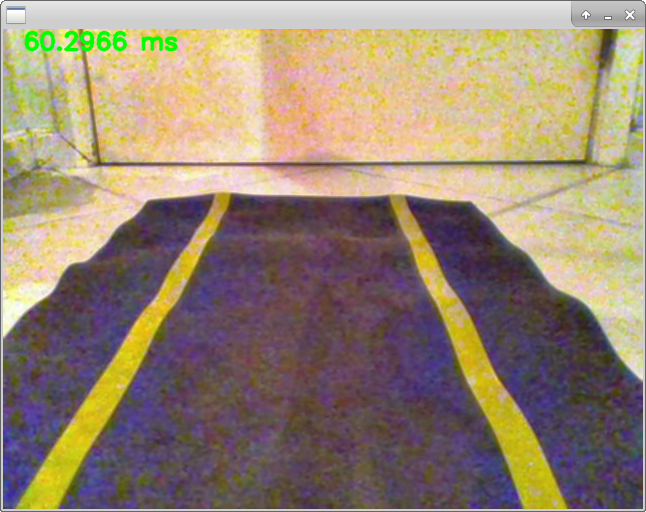2. Equalization
Used to normalize the brightness of the pixels in the image. A bright image will have all pixels confined to high values. In order to have a good image, the equalization stretches these values across the image. This improves the contrast of the image.
3. Filter Colors
Colors are filtered to obtain only the yellow tones of the frame.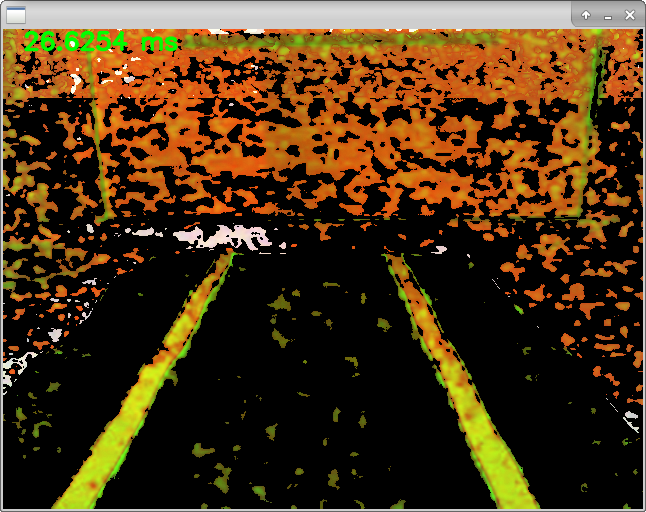4. Canny
Finds the edges of the input image and and marks them in the output map edges using the Canny algorithm. The smallest value between thresholds is used for edge linking.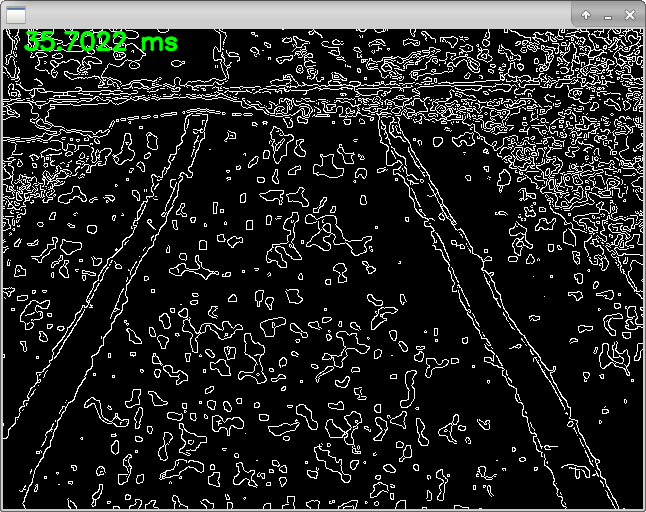5. Probabilistic Hough Transform
The Hough transform is a technique which can be used to isolate features of a particular shape within an image. Because it requires that the desired features be specified in some parametric form, the classical Hough transform is most commonly used for the detection of regular curves such as lines, circles, ellipses, etc. Probabilistic Hough Transform is an optimization of Hough Transform. It doesn’t take all the points into consideration, instead take only a random subset of points and that is sufficient for line detection. Just we have to decrease the threshold.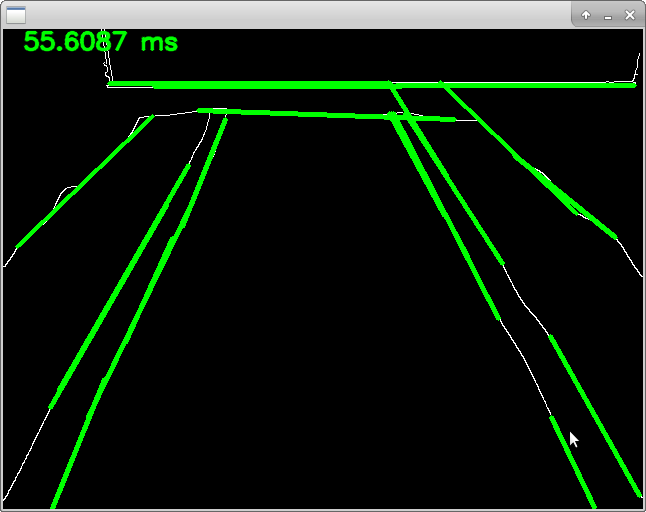## Line Detection Algorithm

```Vec4d LineDetector::detectLine(frame_ref src, frame_ref dest) {

GaussianBlur(src, src, Size(Settings::kernelSize, Settings::kernelSize), 4);
Ptr<CLAHE> clahe = createCLAHE(2.);
vector<Mat> channels;
split(src, channels);

for (auto& c : channels) {
clahe->apply(c, c);
}
merge(channels, src);
Mat gray(src.size(), CV_8UC1);
filterColors(src, gray);
Canny(gray, mask, Settings::lowThreshold, Settings::highThreshold, 3, true);
vector<Vec4i> lines;
HoughLinesP(mask, lines, Settings::rho, Settings::theta, Settings::houghThreshold, Settings::minLineLenght, Settings::maxLineGap);

//here is where the direction algorithm, which will be shown below
}```

## Hardware Software Integration

```#include "_Controller.h"
namespace Rpicar {
_Controller::_Controller() {
wiringPiSetupGpio();
pinMode(BACK_MOTOR_DATA_ONE, OUTPUT);
pinMode(BACK_MOTOR_DATA_TWO, OUTPUT);
pinMode(FRONT_MOTOR_DATA_ONE, OUTPUT);
pinMode(FRONT_MOTOR_DATA_TWO, OUTPUT);
pwmSetMode(PWM_MODE_BAL);
pinMode(BACK_MOTOR_ENABLE_PIN, PWM_OUTPUT);
}

_Controller &_Controller::forward(int velocity) {
assert((uint) velocity <= MAX_PWM_FREQUENCY);
digitalWrite(BACK_MOTOR_DATA_ONE, HIGH);
digitalWrite(BACK_MOTOR_DATA_TWO, LOW);
//pwmWrite(BACK_MOTOR_ENABLE_PIN, velocity);
pwmSetClock(velocity);
return *this;
}

_Controller &_Controller::right() {
digitalWrite(FRONT_MOTOR_DATA_ONE, LOW);
digitalWrite(FRONT_MOTOR_DATA_TWO, HIGH);
return *this;
}

_Controller &_Controller::left() {
digitalWrite(FRONT_MOTOR_DATA_ONE, HIGH);
digitalWrite(FRONT_MOTOR_DATA_TWO, LOW);
return *this;
}

_Controller &_Controller::reverse(int velocity) {
assert((uint) velocity <= MAX_PWM_FREQUENCY);
digitalWrite(BACK_MOTOR_DATA_ONE, LOW);
digitalWrite(BACK_MOTOR_DATA_TWO, HIGH);
pwmWrite(BACK_MOTOR_ENABLE_PIN, velocity);
return *this;
}

_Controller& _Controller::stop() {
return this->idle(Motor::BOTH);
}

_Controller& _Controller::after(uint milliseconds) {
usleep(milliseconds * 1000);
return *this;
}

_Controller &_Controller::idle(Motor which) {
int modeBack = ((int)which) & 1, modeFront = (((int)which) >> 1) & 1;
digitalWrite(BACK_MOTOR_DATA_ONE, modeBack);
digitalWrite(BACK_MOTOR_DATA_TWO, modeBack);
digitalWrite(FRONT_MOTOR_DATA_ONE, modeFront);
digitalWrite(FRONT_MOTOR_DATA_TWO, modeFront);
return *this;
}

_Controller &_Controller::operator<<(const Vector &direction) {
int velocity = MAX_PWM_FREQUENCY;//(int) floor(direction.length());

if (direction.length() == 0) {
this->stop();
return *this;
}
// Y axis
if (direction.y() == 0)
this->idle(BACK);
else if (direction.y() > 0)
this->forward(velocity);
else if (direction.y() < 0)
this->reverse(velocity);
if (direction.x() == 0)
this->idle(FRONT);
else if (direction.x() > 0)
this->right();
else if (direction.x() < 0)

// X axis
this->left();

return *this;
}
//pwm issues - api supports
_Controller& _Controller::operator<<(const Wait &wait) {
this->after(wait.milliseconds); //milliseconds the controller has to wait
return *this;
}

Vector::Vector(double x, double y) {
this->_x = x;
this->_y = y;
this->_length = sqrt(pow(this->_x, 2) + pow(this->_y, 2));
this->_slope = x ? y / x : 0.;
}

Vector &Vector::rotate(double angle) const {
angle *= (M_PI/180.0);
double s = sin(angle), c = cos(angle);
return *(new Vector(c * this->_x - s * this->_y, s * this->_x + c * this->_y));
}

Vector &Vector::normal() const {
return *(new Vector(this->_x / this->_length, this->_y / this->_length));
}

Vector &Vector::operator-() const {
return *(new Vector(-this->_x, -this->_y));
}

Vector &Vector::operator+(const Vector &other) const {
return *(new Vector(this->_x + other._x, this->_y + other._y));
}

Vector &Vector::operator*(double scalar) const {
return *(new Vector(scalar * this->_x, scalar * this->_y));
}
}
/*
Region of Interest(ROI) - One of the approaches taken was to extract the roi of the image, that is cutting the frame in a trapezium. This is an attempt to get as minimum interference from external sources as possible in the region that the camera is pointed.
*/
void LineDetector::extractROI(frame_ref src, frame_ref dest) {
Mat mask = Mat::zeros(src.size(), CV_8UC1);
int h = src.size().height, w = src.size().width;
int topWidth = cvFloor(w * .7),
bottomWidth = cvFloor(w * .85),
height = cvFloor(h * .4);
int yTop = (h - height) >> 1,
gapTop = (w - topWidth) >> 1,
gapBottom = (w - bottomWidth) >> 1;
vector<Point> points({Point(gapBottom, h), Point(w-gapBottom, h),
Point(w-gapTop, yTop), Point(gapTop, yTop)});
fillConvexPoly(mask, points, Scalar(255, 255, 255));
bitwise_and(src, src, dest, mask);
}

/*
Detecting lines and direction - There are multiple lines detected on the frame. Without considering the interference from external sources(lighting, reflection, irregular surface), we are able to determine the slope and length of the lines traced.
We want one lane line. When the car has to turn left, the lines slope will be positive, and if the slope is negative, the car has to turn right. Many variables have to be considered when a direction of turning has to be chosen, for instance the current car speed, the external interference, and the response time for processing a frame. This is currently the biggest challenge we faced.
*/
HoughLinesP(mask, lines, Settings::rho, Settings::theta, Settings::houghThreshold, Settings::minLineLenght, Settings::maxLineGap);
Vector direction;
int c = 0;
for (auto v : lines) {
Vector vc(v);
if (vc.slope() > 0.) {
//    line(dest, Point(v, v), Point(v, v), Scalar(0, 255, 0), 3);
direction = direction + vc;
++c;
}
}
if(c) {
direction = direction * (1./(double)c);
Point s(dest.size().width >> 1, dest.size().height);
line(dest, s, Point(s.x + direction.x(), s.y - direction.y()), Scalar(0, 255, 0), 3);
}```

## Direction decision

To decide the direction, we make the arithmetic mean of the vectors drawn in the previous steps.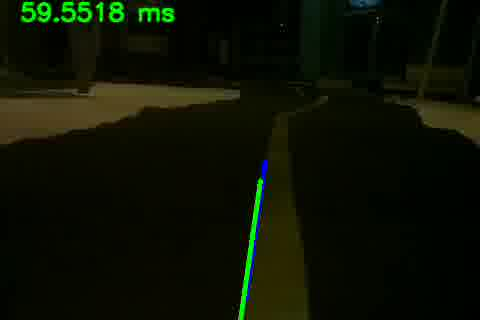In this case it was detected only one vector (blue line), so its mean is itself (green line).

## Difficulties faced

The processing power of the Raspberry Pi is limited, so we had problems using the filter colors because the image processing got extremely slow. We decided to remove this filter, but it started to draw lines beyond those we wanted.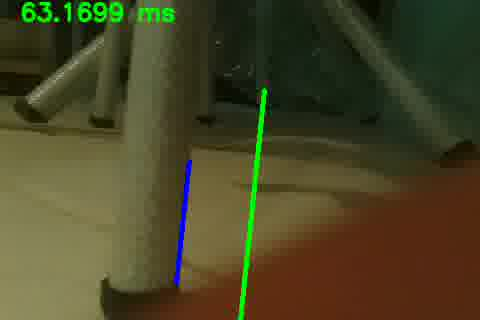## Bibliography

1. https://github.com/samjabrahams/tensorflow-on-raspberry-pi
2. GARCIA, G.B.; SUAREZ, O.D.; ARANDA, J.L.E.; Learning Image Processing With OpenCV. Birmingham: Packt, 2015. 208p.
3. OpenCV Neural Network Documentation: http://docs.opencv.org/2.4/modules/ml/doc/neural_networks.html
4. OpenCV Documentation: http://docs.opencv.org/2.4/modules/refman.html
5. L293D Datasheet. Texas Instruments, 1998.
6. PFRETZCHNER, B. Autonomous Car Driving Using a Low-Cost On-Board Computer. 2013. 64p. Bachelor of Science. Department of Computer Science, Technische Universitat Darmtast. June 30, 2013.
7. PANNU, G.S.; ANSARI, M.D.; GUPTA, P.; Design and Implementation of Autonomous Car Using Raspberry Pi. International Journal of Computer Applications, 2015. Vol.113.
8. https://github.com/multunus/autonomous-rc-car